# 2.2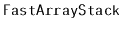: An Optimized ArrayStack

Much of the work done by an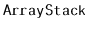involves shifting (byand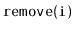) and copying (by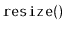) of data. In the implementations shown above, this was done usingloops. It turns out that many programming environments have specific functions that are very efficient at copying and moving blocks of data. In the C programming language, there are the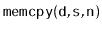and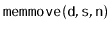functions. In the C++ language there is the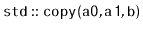algorithm. In Java there is the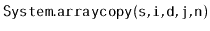method.

```  void resize() {
array<T> b(max(1, 2*n));
std::copy(a+0, a+n, b+0);
a = b;
}
void add(int i, T x) {
if (n + 1 > a.length) resize();
std::copy_backward(a+i, a+n, a+n+1);
a[i] = x;
n++;
}
```

These functions are usually highly optimized and may even use special machine instructions that can do this copying much faster than we could by using a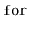loop. Although using these functions does not asymptotically decrease the running times, it can still be a worthwhile optimization.

In the C++ implementations here, the use of the nativeresulted in speedups of a factor between 2 and 3, depending on the types of operations performed. Your mileage may vary.

opendatastructures.org